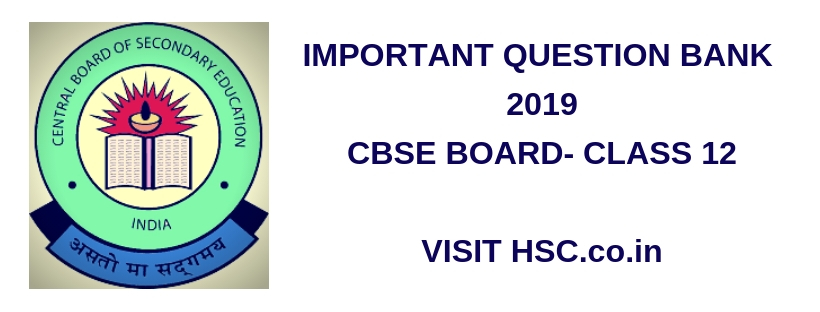# Mathematics – Important Question Bank for CBSE Class XII (HSC) Board Exam 2019

HSC Board Exams are fast approaching and students are getting anxious about how to prepare for their HSC Board Exams. So we had mentioned some

After the tremendous success of Important Questions Bank HSC (12th Std) Board Exam 2016 and 2017 we have also created a list ofMost Important Questions Bank for CBSE Class XII (HSC) Board Exam 2019 which are likely to appear in HSC Board Exams this year.To unlock the content Click on any of 3 buttons available in the box below saying “This content is Locked”. Once you click on the button the content will get unlocked on same page itself. You must click on social media button showing in below box ie Facebook, Twitter or Google Plus to unlock the content.

1. If x+y=, then write the value of x.
2. Two cards are drawn successively without replacement from a well shuffles deck of cards. Find the mean and standard variation of the random variable X where X is the number of aces.
3. Evaluate4. Assume that each born child is equally like to be a boy or a girl. If a family has two children, what is the conditional probability that both are girls given that (i) the youngest is a girl. (ii) at least one is a girl?
5. Maximize Z=3x+4.
Subject to the constraints: x+y<4, x>0 , y>0.
6. Write a vector in the direction of the vectorthat has magnitude of 9 units.
7. Is h(x) = (5×2 + 7x + 2) / x3 + x2 + x + 3 a continuity function?
8. Find the adjoint of.
9. A bag contains 5 red marbles and 3 black marbles. Three marbles are drawn one by one without replacement. What is the probability that at least on of the three marbles drawn be black, if the first marble is red?
10. Evaluate11. Derive the condition for the co planarity of two lines in space both in the vector form and Cartesian form.
12. Differentiate the function with respect to13. Solve the following system of equations by matrix method,
3x-2y+3z=8 ; 2x+y-z=1 and 4x-3y+2z=4.
14. Show that15. Maximize Z= -x+2y, subject to the constraints: x>2, x+y>5 ,y>0.
16. Explain why the experiment of tossing a coin three times is said to have a binomial distribution.
17. Differentiate with respect to y = (5x – 3) ^ x
18. Evaluate the determinants of.

Hi, we’re trying to collate and gather the data and would be updating it here a few days before the exam. Please keep on visiting our website for updates.

Please use the comments box below and post questions that you think are important from your analysis. It would help the HSC community a lot.

Do subscribe to our updates so that you do not miss out on any important information that we push your way.

Don’t forget to read : MUST REMEMBER THINGS on the day of Exam for HSC Students